### PI Function Examples – Excel, VBA, & Google Sheets

This tutorial demonstrates how to use the Excel PI Function in Excel to return the value of PI.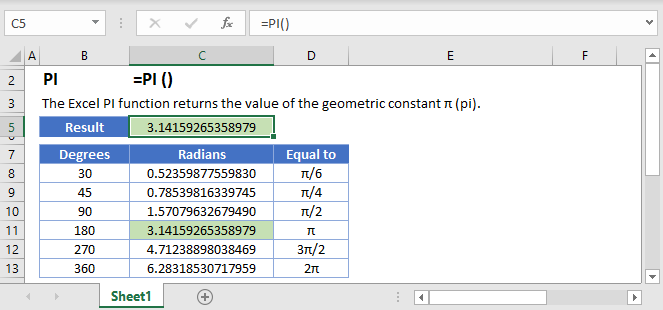## PI Function OVERVIEW

The PI function returns the value of π , 3.14159265358979, accurate to 15 digits.

To use the PI Excel Worksheet Function, select a cell and type: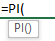(Notice how the formula inputs appear)

### PI Function Syntax and Inputs:

``=PI()``
AutoMacro - VBA Code Generator

### Decimal places

When using the PI function, Excel stores the value to 15 decimal places. To show more or less decimal places, use the Increase Decimal or Decrease Decimal button in the Home menu.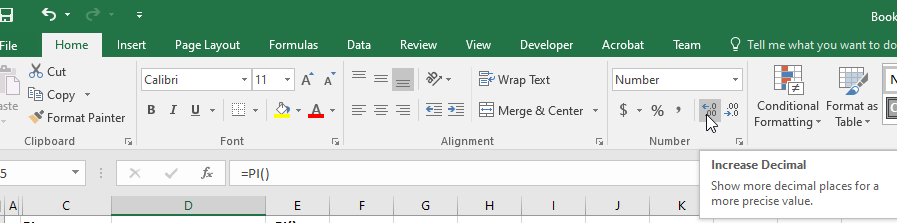Because π is only calculated to 15 decimal places, exact values for trigonometric functions are not always returned. See more details in the SIN, COS, and TAN functions. <insert links to each of these pages>

### Converting to degrees

To convert the value of π to degrees, use the function

`=DEGREES(PI())`### Common Errors

When calculating scalar multiples of π , the operation symbol (like multiply or divide) is necessary. Without it, the following error is displayed.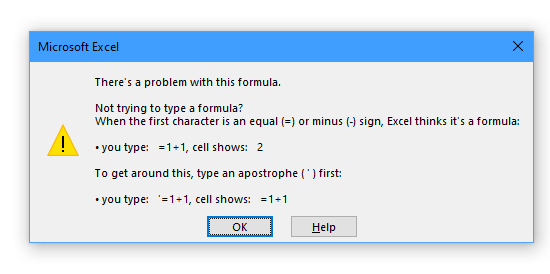## PI in Google Sheets

The PI Function works exactly the same in Google Sheets as in Excel: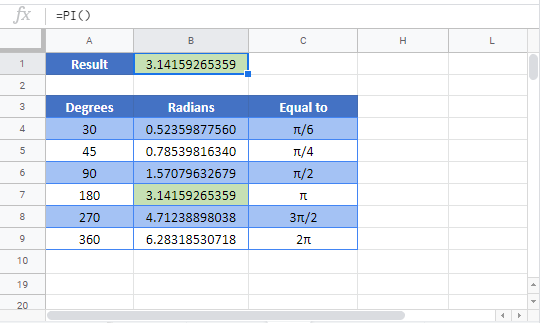## PI Examples in VBA

You can also use the PI function in VBA. Type:
`application.worksheetfunction.pi()`

Executing the following VBA statement

`Range("A1") = Application.WorksheetFunction.Pi()`

will produce the following result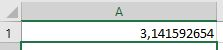For the function arguments (, etc.), you can either enter them directly into the function, or define variables to use instead.

Return to the List of all Functions in Excel

For more information about the PI Formula visit the
Microsoft Website.

## Excel Practice Worksheet

Practice Excel functions and formulas with our 100% free practice worksheets!

• Automatically Graded Exercises
• Learn Excel, Inside Excel!